# HSSlive: Plus One & Plus Two Notes & Solutions for Kerala State Board

## BSEB Class 10 Maths Chapter 2 Polynomials Ex 2.2 Textbook Solutions PDF: Download Bihar Board STD 10th Maths Chapter 2 Polynomials Ex 2.2 Book AnswersBSEB Class 10 Maths Chapter 2 Polynomials Ex 2.2 Textbook Solutions PDF: Download Bihar Board STD 10th Maths Chapter 2 Polynomials Ex 2.2 Book Answers

BSEB Class 10th Maths Chapter 2 Polynomials Ex 2.2 Textbooks Solutions and answers for students are now available in pdf format. Bihar Board Class 10th Maths Chapter 2 Polynomials Ex 2.2 Book answers and solutions are one of the most important study materials for any student. The Bihar Board Class 10th Maths Chapter 2 Polynomials Ex 2.2 books are published by the Bihar Board Publishers. These Bihar Board Class 10th Maths Chapter 2 Polynomials Ex 2.2 textbooks are prepared by a group of expert faculty members. Students can download these BSEB STD 10th Maths Chapter 2 Polynomials Ex 2.2 book solutions pdf online from this page.

## Bihar Board Class 10th Maths Chapter 2 Polynomials Ex 2.2 Books Solutions

 Board BSEB Materials Textbook Solutions/Guide Format DOC/PDF Class 10th Subject Maths Chapter 2 Polynomials Ex 2.2 Chapters All Provider Hsslive

## How to download Bihar Board Class 10th Maths Chapter 2 Polynomials Ex 2.2 Textbook Solutions Answers PDF Online?

2. Click on the Bihar Board Class 10th Maths Chapter 2 Polynomials Ex 2.2 Answers.
3. Look for your Bihar Board STD 10th Maths Chapter 2 Polynomials Ex 2.2 Textbooks PDF.
4. Now download or read the Bihar Board Class 10th Maths Chapter 2 Polynomials Ex 2.2 Textbook Solutions for PDF Free.

Find below the list of all BSEB Class 10th Maths Chapter 2 Polynomials Ex 2.2 Textbook Solutions for PDF’s for you to download and prepare for the upcoming exams:

## BSEB Bihar Board Class 10th Maths Solutions Chapter 2 Polynomials Ex 2.2

Question 1.
Find the zeroes of the following quadratic polynomials and verify the relationship between the zeroes and the coefficients:

1. x2 – 2x – 8
2. 4s2 – 4s + 1
3. 6x2 – 3 – 7x
4. 4u2 + 8u
5. t2 – 15
6. 3x2 – x – 4

Solution:
1. We have:
x2 – 2x – 8 = x2 + 2 – 4x – 8
= x(x + 2) – 4(x + 2)
= (x + 2)(x – 4)
The value of x2 – 2x – 8 is 0, when the value of (x + 2)(x – 4) is 0, i.e., when x + 2 = 0 or x – 4 = 0, i.e., when x = – 2 or x = 4.
∴ The zeroes of x2 – 2x – 8 are – 2 and 4.
Therefore, sum of the zeroes = (- 2) + 4 = 2 = −(−2)1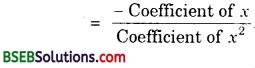and product of zeroes = (- 2)(4) = – 8 = −81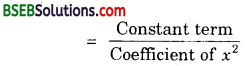2. We have:
4s2 – 4s + 1 = 4s2 – 2s – 2s + 1
= 2s(2s – 1) – 1(2s – 1)
= (2s – 1)(2s – 1)
The value of 4s2 – 4s + 1 is 0, when the value of (2s – 1)(2s – 1) is 0, i.e., when 2s – 1 = 0 or 2s – 1 = 0, i.e.,
when s = 12 or s = 12.
∴ The zeroes of 4s2 – 4s + 1 are 12 and 12.
Therefore, sum of the zeroes = 12 + 12 = 1 = −(−4)4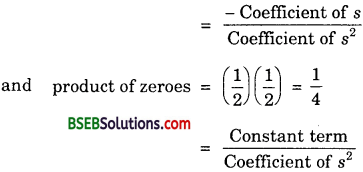3. We have:
6x2 – 3 – 7x = 6x2 – 7x – 3
= 6x2 – 9x + 2x – 3
= 3x(2x – 3) + 1(2x – 3)
= (3x + 1)(2x – 3)
The value of 6x2 – 3 – 7x is 0, when the value of (3x + 1)(2x – 3) is 0, i.e; when 3x + 1 = 0 or 2x – 3 = 0, i.e;
when x = – 13 or x = 32.
∴ The zeros of 6x2 – 3 – 7x are – 13 and 32.
Therefore, sum of the zeros = – 13 + 32 = 76 = −(−7)64. We have:
4u2 + 8u is 0, when the value of 4u(u + 2) is 0, i.e; when u = 0 or u + 2 = 0, i.e; when u = 0 or u = – 2.
∴ The zeroes of 4u2 + 8u are o and – 2.
Therefore, sum of the zeroes = 0 + (- 2) = – 2 = −84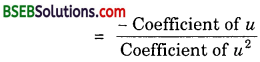and product of zeroes = (0)(- 2) = 0 = 04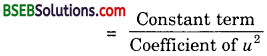5. We have:
t – 15 = (t – 15‾‾‾√)(t + 15‾‾‾√)
The value of t2 – 15 is 0, when the value of (t – 15‾‾‾√)(t + 15‾‾‾√) is 0, i.e; when t – 15‾‾‾√ = 0 or t + 15‾‾‾√ = 0,
i.e; when t = 15‾‾‾√ or t = – 15‾‾‾√.
∴ The zeroes of t2 – 15 are 15‾‾‾√ and – 15‾‾‾√.
Therefore, sum of the zeroes = 15‾‾‾√ + (- 15‾‾‾√) = 0and product of the zeroes = (15‾‾‾√)(-15‾‾‾√)
= – 15 = −1516. We have:
3x2 – x – 4 = 3x2 + 3x – 4x – 4
= 3x(x + 1) – 4(x + 1)
= (x + 1)(3x – 4)
The value of 3x2 – x – 4 is 0, when the value of (x + 1)(3x – 4) is 0, i.e; when x + 1 = 0 or 3x – 4 = 0, i,e; when x = – 1 or x = 43.
∴ The zeroes of 3x2 – x – 4 are – 1 and 43.
Therefore, sum of the zeroes = – 1 + 43 = −3+43
= 13 = −(−1)3and product of the zeroes = (- 1)(43) = – 43 = −43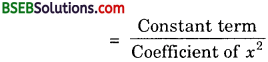Question 2.
Find a quadratie polynomial each with the given numbers as the sum and product of its zeroes respectively.

1. 14, – 1
2. 2‾√, 13
3. 0, 5‾√
4. 1, 1
5. – 14, 14
6. 4, 1

Solution:
1. Let the polynomial be ax2 + bx + c, and its zeroes be α and β. Then,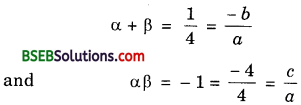If a = 4, then b = – 1 and c = – 4.
∴ One quadratic polynomial which fits the given conditions is 4x2 – x – 4.

2. Let the polynomial be ax2 + bx + c, and its zeroes be α and β. Then,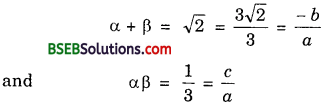If a = 3, then b = – 32‾√ and c = 1.
∴ One quadratic polynomial which fits the given conditions is 3x2 – 32𝑥‾‾‾√ + 1.

3. Let the polynomial be ax2 + bx + c, and its zeroes be α and β. Then,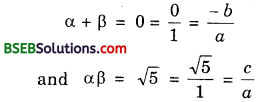If a = 1, then b = 0 and c = 5‾√.
∴ One quadratic polynomial which fits the given conditions is x2 – 0. x + 5‾√, i.e; x2 + 5‾√.

4. Let the polynomial be ax2 + bx + c, and its zeroes be α and β. Then,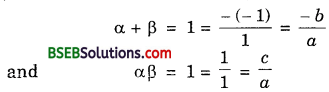If a = 1, then b = – 1 and c = 1.
∴ One quadratic polynomial which fits the given conditions is x2 – x + 1.

5. Let the polynomial be ax2 + bx + c and its zeroes be α and β. Then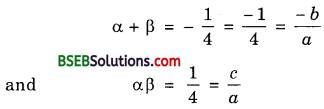If a = 4, then b = 1 and c = 1.
∴ One quadratic polynomial which fits the given conditions is 4x2 + x + 1.

6. Let the polynomial be ax2 + bx + c and its zeroes be α and β. Then,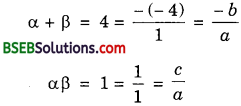If a = 1, then b = – 4, and c = 1.
∴ One quadratic polynomial which fits the given conditions is x2 – 4x + 1.

## Bihar Board Class 10th Maths Chapter 2 Polynomials Ex 2.2 Textbooks for Exam Preparations

Bihar Board Class 10th Maths Chapter 2 Polynomials Ex 2.2 Textbook Solutions can be of great help in your Bihar Board Class 10th Maths Chapter 2 Polynomials Ex 2.2 exam preparation. The BSEB STD 10th Maths Chapter 2 Polynomials Ex 2.2 Textbooks study material, used with the English medium textbooks, can help you complete the entire Class 10th Maths Chapter 2 Polynomials Ex 2.2 Books State Board syllabus with maximum efficiency.

## FAQs Regarding Bihar Board Class 10th Maths Chapter 2 Polynomials Ex 2.2 Textbook Solutions

#### Can we get a Bihar Board Book PDF for all Classes?

Yes you can get Bihar Board Text Book PDF for all classes using the links provided in the above article.

## Important Terms

Bihar Board Class 10th Maths Chapter 2 Polynomials Ex 2.2, BSEB Class 10th Maths Chapter 2 Polynomials Ex 2.2 Textbooks, Bihar Board Class 10th Maths Chapter 2 Polynomials Ex 2.2, Bihar Board Class 10th Maths Chapter 2 Polynomials Ex 2.2 Textbook solutions, BSEB Class 10th Maths Chapter 2 Polynomials Ex 2.2 Textbooks Solutions, Bihar Board STD 10th Maths Chapter 2 Polynomials Ex 2.2, BSEB STD 10th Maths Chapter 2 Polynomials Ex 2.2 Textbooks, Bihar Board STD 10th Maths Chapter 2 Polynomials Ex 2.2, Bihar Board STD 10th Maths Chapter 2 Polynomials Ex 2.2 Textbook solutions, BSEB STD 10th Maths Chapter 2 Polynomials Ex 2.2 Textbooks Solutions,
Share: